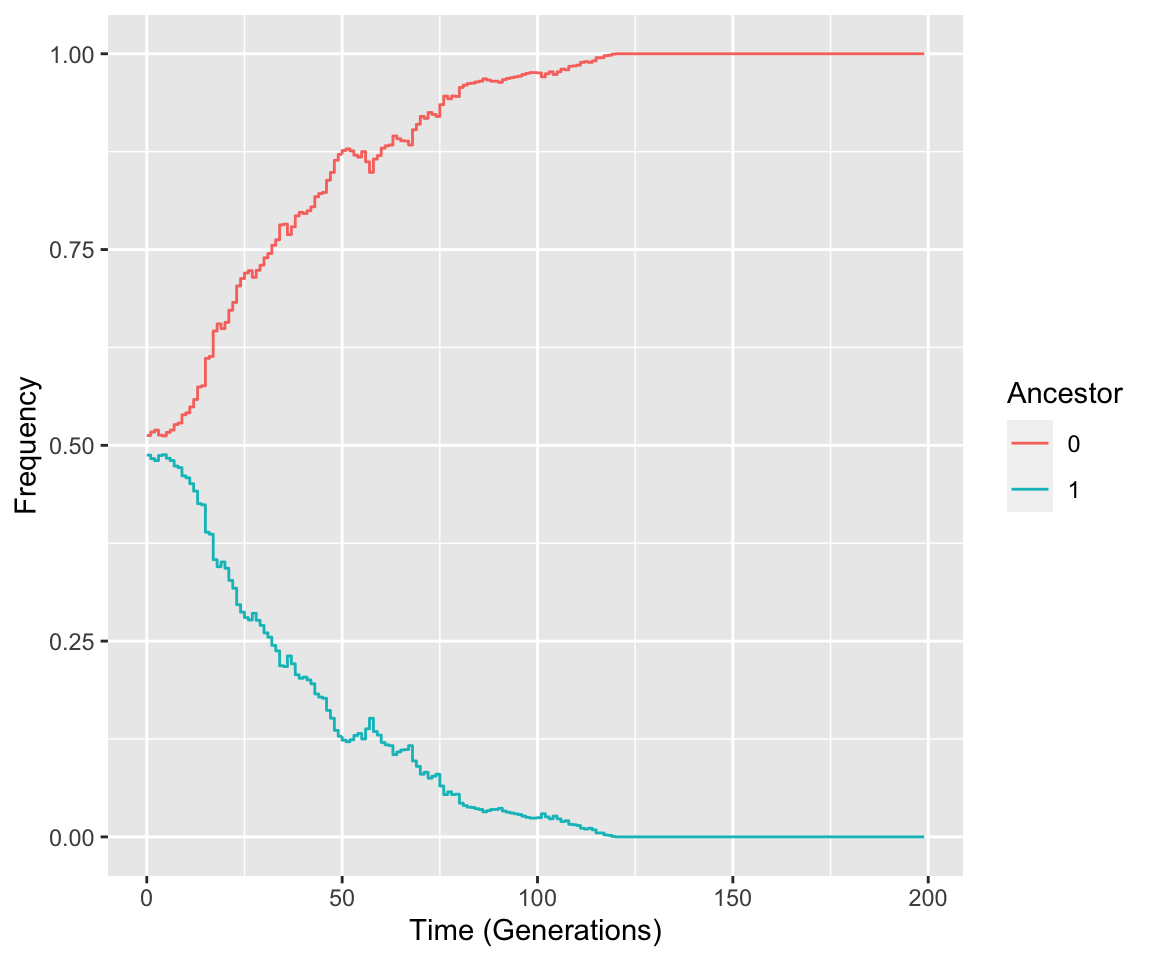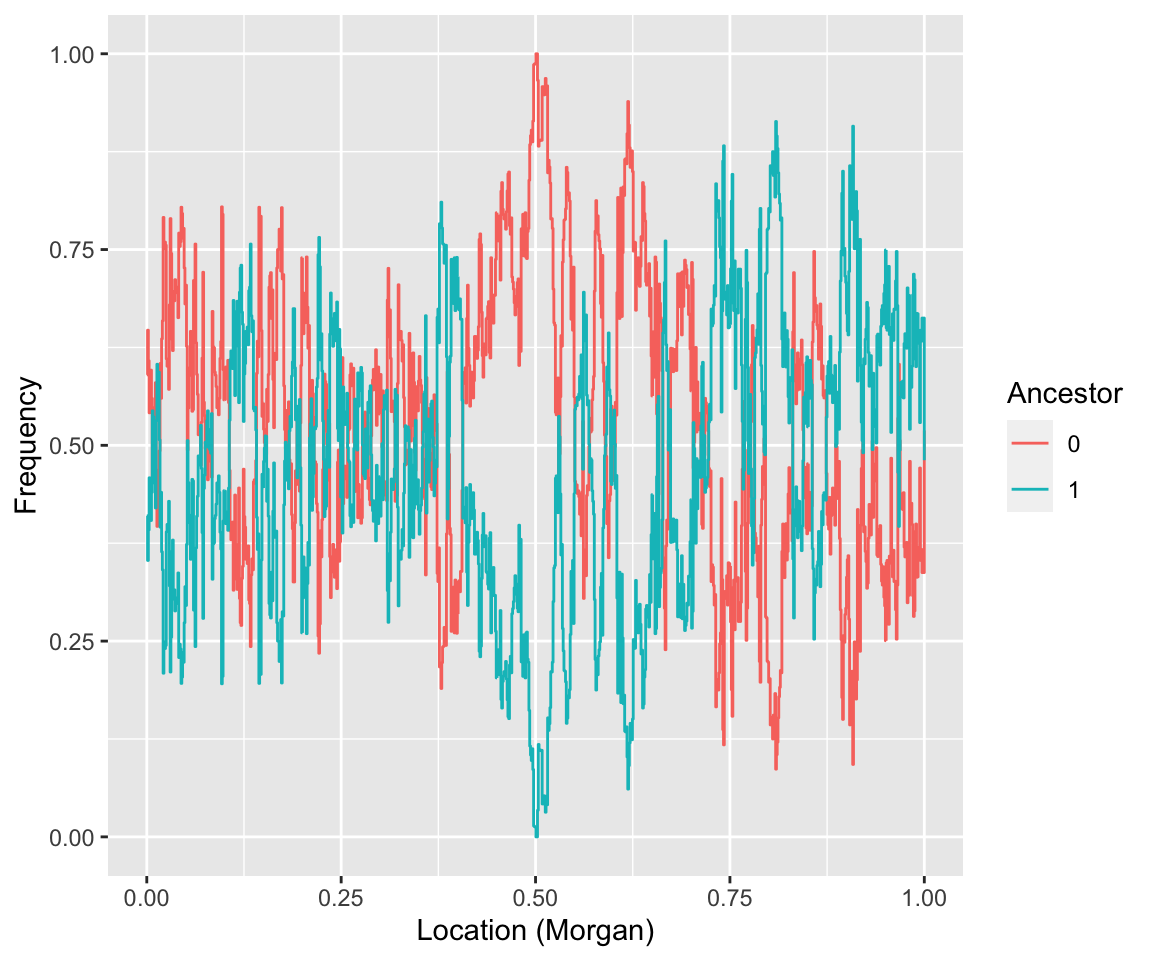# Visualization

The GenomeAdmixR package provides many different visualization options, here we will explore a variety of them. First, we simulate a scenario with selection, to obtain somewhat meaningful results.

``````select_matrix <- matrix(nrow = 1, ncol = 5)
select_matrix[1, ] <- c(0.5, 1, 1 + 0.05, 1 + 0.1, 0)
module = ancestry_module(markers =
c(0.5,
seq(0, 1, length.out = 100))),
pop_size = 1000,
total_runtime = 200,
select_matrix = select_matrix)``````
``## Found a selection matrix, performing simulation including selection``

Now, we can first view whether selection on our marker has yielded an increase in frequency:

## Plot over time

``plot_over_time(population\$frequencies, focal_location = 0.500)``Indeed, we observe that over time the frequency of the allele under selection (0) increases to fixation due to selection.

## Plot Frequencies

How are the alleles scattered across the genome? we can answer that with the function plot_frequencies:

``plot_frequencies(population, locations = seq(0, 1, length.out = 1000))``As expected, we observe a huge increase around the location of the marker under selection (at 0.5 Morgan).

## plot difference frequencies

If instead, we are interested in the change in frequency of a marker, we can do so using plot_difference_frequencies.

``plot_difference_frequencies(population)``